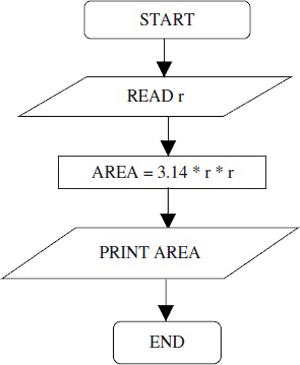# Quick Review: Graphs and its Types

Graphs form an essential part of Data Interpretation. You need to be aware of the different kinds of graphs to handle DI questions accurately.

###### Types of Graphs
1. Pie Chart/Circle Graph: As the word suggests, a circle graph is shaped like a circle. It is divided into fractions that resemble the pieces of a pie. Because of this reason, a circle graph is also known as a pie graph.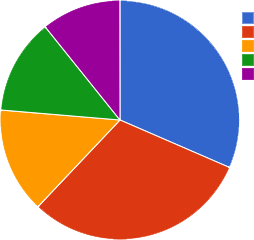2. Bar Graph: A bar graph represents data by using graphs. The bars can be either be vertical or horizontal. The data can be in words or numbers.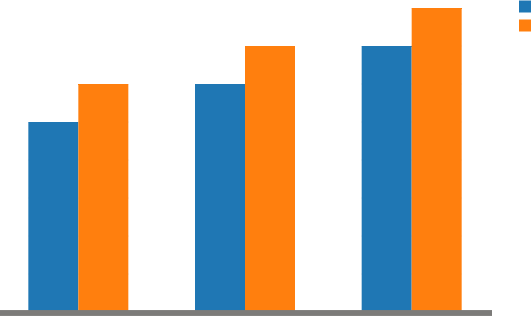3. Histogram Graph: A graphical display of data using bars of different heights is known as a histogram graph.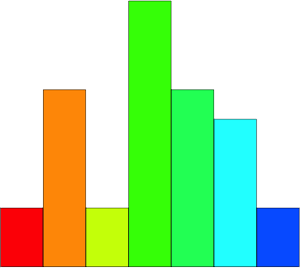4. Line Graph: A line graph shows points plotted on a graph. These points are connected to form a line.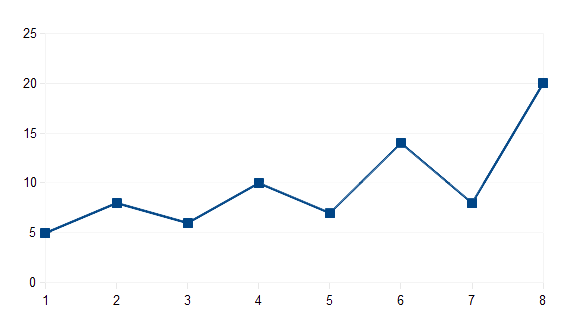5. Flowcharts: Flowcharts display a schematic process. Many companies use flowcharts to depict all the stages of a project. It helps to breakdown the process and understand the whole scenario.